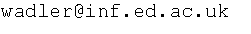# Parametricity

### The Girard-Reynolds isomorphism (second edition)

Philip Wadler. Theoretical Computer Science, 375(1–3):201–226, May 2007. Festschrift for John C. Reynolds's 70th birthday.

Jean-Yves Girard and John Reynolds independently discovered the second-order polymorphic lambda calculus, F2. Girard additionally proved a Representation Theorem: every function on natural numbers that can be proved total in second-order intuitionistic predicate logic, P2, can be represented in F2. Reynolds additionally proved an Abstraction Theorem: every term in F2 satisfies a suitable notion of logical relation; and formulated a notion of parametricity satisfied by well-behaved models.

We observe that the essence of Girard's result is a projection from P2 into F2, and that the essence of Reynolds's result is an embedding of F2 into P2, and that the Reynolds embedding followed by the Girard projection is the identity. We show that the inductive naturals are exactly those values of type natural that satisfy Reynolds's notion of parametricity, and as a consequence characterize situations in which the Girard projection followed by the Reynolds embedding is also the identity.

An earlier version of this paper used a logic over untyped terms. This version uses a logic over typed terms, similar to ones considered by Abadi and Plotkin and Takeuti, which better clarifies the relationship between F2 and P2.

### The Girard-Reynolds isomorphism

Philip Wadler. Theoretical Aspects of Computer Software, Sendai, Japan, October 2001. Journal version in Information and Computation, 186:260-284, 2003.

The second-order polymorphic lambda calculus (F2) was independently discovered by Girard and Reynolds. Girard additionally proved a representation theorem: every function on natural numbers that can be proved total in second-order propositional logic (P2) can be represented in F2. Reynolds additionally proved an abstraction theorem: for a suitable notion of logical relation, every term in F2 takes related arguments into related results. We observe that the essence of Girard's result is a projection from P2 into F2, and that the essence of Reynolds's result is an embedding of F2 into P2, and that the Reynolds embedding followed by the Girard projection is the identity. The Girard projection discards all first-order quantifiers, so it seems unreasonable to expect that the Girard projection followed by the Reynolds embedding should also be the identity. However, we show that in the presence of Reynolds's parametricity property that this is indeed the case, for propositions corresponding to inductive definitions of the naturals. The result extends to other algebraic types.

#### Information and Computation version available in: preprint pdf, I&C web site.

This note discusses encodings of least and greatest fixpoints in polymorphic lambda calculus. It is shown that the encoding of the least fixpoint is a weakly initial algebra, and is an initial algebra exactly when parametricity holds at that type. Parametricity is also used to show the equivalence of iterators and recursors. Finally, the dual development for greatest fixpoints is presented.

#### Available in: txt.

Philip Wadler,